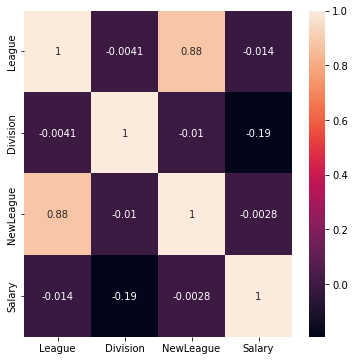# Prediction using Lasso and Ridge Regression

## Introduction

In this blog, we have discussed how to implement the prediction using the lasso and Ridge regression algorithm. From the previous blog, we know that “lasso and ridge regression” adds the penalty factor to the Linear regression cost function, ie MSE to avoid overfitting or underfitting. From the sklearn library, we use the inbuilt linear regression function for prediction.

1. Numpy
2. Pandas
3. Matplotlib
4. Seaborn
5. sklearn

## Dataset

This data set was originally obtained from the Carnegie Mellon University-maintained StatLib library. This is a portion of the data that was used in the poster session for the 1988 ASA Graphics Section. The salary information was first published in Sports Illustrated on April 20, 1987. The 1987 Baseball Encyclopedia Update, published by Collier Books, Macmillan Publishing Company, New York, provided the career statistics.

## Implementation of Hitters Salary prediction using Lasso and Ridge Regression

### Step-1: Importing Libraries and dataset

Firstly, we need to import the required libraries and datasets. Dataset can be downloaded at hitters.csv

# Importing necessary libraries

import numpy as np
import pandas as pd

# importing visualizing libraries

import seaborn as sns
import matplotlib.pyplot as plt

# displays the first 5 rows of the dataset
df = pd.DataFrame(data)



### Step-2: Preprocessing the data

We check for any null values in the dataset. If there are more Not available values in the dataset we remove that column.

# display column wise percentage of NaN values

for i in col:
print(i,"\t-\t", df[i].isna().mean()*100)

Since the given dataset contains both categorical and numerical variables we have to separate them for further analysis.

# numerical variables stored in num_df
num_df = df.select_dtypes(exclude=['object'])

# categorical variables stored in cat_df
cat_df= df.drop(num_df, axis=1)

Next, we search for the top most correlated attributes because the variables having less correlation contribute very less towards the prediction.

def get_corelated_col(cor_dat, threshold):
# Cor_data to be column along which corelation to be measured
#Threshold be the value above wich of corelation to considered

feature=[]
value=[]

for i ,index in enumerate(cor_dat.index):
if abs(cor_dat[index]) > threshold:
feature.append(index)
value.append(cor_dat[index])

df = pd.DataFrame(data = value, index = feature, columns=['corr value'])
return df

# we get the co-relation values having greater than 0.4
top_corelated_values = get_corelated_col(cormap['Salary'],0.40)

# save the columns of having high correlation value
final_num_df = num[top_corelated_value.index]
Now, let us deal with the categorical variables. We turn the categorical variables into 0 and 1.
# We import labelencoder module from sklearn
from sklearn.preprocessing import LabelEncoder

# get the columns of categorial variables
cat_col = cat_df.columns

# for all columns in categorical dataset
for i in cat_col:
enc = LabelEncoder()
# transform into numerical variables
cat_df[i] = enc.fit_transform(cat_df[i].astype('str'))

# get coreltion with target attribute which is Sales Price
cat_df['Salary'] = df['Salary']

# get the co-relation and plot it using seaborn library

cormat = cat_df.corr()
fig, ax = plt.subplots(figsize=(6,6))
sns.heatmap(cormat, annot = True)The above image is the output of the correlation of categorical variables. Since no columns have appreciable co-relation, we are not using categorical collums for predictions.

### Step-3: Splitting the dataset.

X is set to all the columns except the Salary column and Y is set to the Salary column which we are going to predict.

# Here we are splitting data in train and test as test set are rows with null values in salary column

df_train = final_df.dropna()
df_test = final_df[final_df.isnull().any(axis=1)]

X = df_train.drop(['Salary'], axis=1)
y = df_train['Salary']

We use the Min-Max scaler method for normalizing the features and variables of the input. The result is that all features will be converted into a range [0,1], with the minimum and maximum values of each feature or variable being 0 and 1, respectively.

# we import minmaxscaler from the sklearn library
from sklearn.preprocessing import MinMaxScaler

scaler = MinMaxScaler()
# we apply minmaxscaler to X
X = pd.DataFrame(scaler.fit_transform(X), columns=X.columns)

# similarly we do it for test data
df_test = pd.dataframe(scaler.transform(df_test.drop(["salary"],axis=1)),columns=X.columns)
we split our variable into training and testing sets. It is usually a good practice to keep 70% of the data in your train dataset and the rest 30% in your test dataset
# we import train_test_split and linear regression module from sklearn

from sklearn.model_selection import train_test_split
from sklearn.linear_model import LinearRegression

X_train, X_test, y_train, y_test = train_test_split(X, y, test_size = 0.3)

### Lasso Regression

We use an inbuilt model from the sklearn library to build the Lasso model and fit our training data to it.

# we choose LassoCV to per 5 fold cross validation on data so that we can get best alpha while training

from sklearn.linear_model import LassoCV

lasso = LassoCV(cv = 5)
lasso.fit(X_train,y_train)

We test the model using the test data that is to predict the salary.

# Predicting Values of df_test using the above trained model

df_pred = lasso.predict(df_test)

### Ridge Regression

Similar to Lasso regression, We use an inbuilt model from the sklearn library to build the Ridge model and fit our training data to it.

# we import RidgeCV function from sklearn library
from sklearn.linear_model import RidgeCV

ridge = RidgeCV(cv = 5)
ridge.fit(X_train,y_train)

We test the model using the test data that is to predict the salary.

# Predicting Values of df_test using the above trained model

df_pred = ridge.predict(df_test)

### Step-5: Evaluation Metrics

Evaluation metrics show how well your model is performing.  We use MAE, MSE, RMSE, and R squared error as our evaluation metrics.

from sklearn import metrics

print('Mean Absolute Error:', metrics.mean_absolute_error(y_test, y_pred_))
print('Mean Squared Error:', metrics.mean_squared_error(y_test, y_pred_))
print('Root Mean Squared Error:', np.sqrt(metrics.mean_squared_error(y_test, y_pred_)))
print('R2 Value:', metrics.r2_score(y_test, y_pred_))